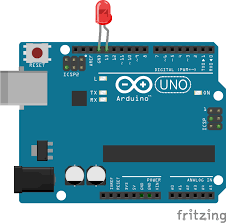# SOS code with simple instructions

This is an Arduino code using simple instructions, that is, digitalWrite in order to switch ON or OFF a LED and delay in order to choose the right time to be ON or OFF:

``````
//This is a SOS code with simple instructions
void setup() {
// initialize digital pin LED_BUILTIN as an output.
pinMode(LED_BUILTIN, OUTPUT);
}

// the loop function runs over and over again forever
void loop() {

digitalWrite(LED_BUILTIN, HIGH);   // turn the LED on (HIGH is the voltage level)
delay(200);                       // wait for a second
digitalWrite(LED_BUILTIN, LOW);    // turn the LED off by making the voltage LOW
delay(200);                       // wait for a second

digitalWrite(LED_BUILTIN, HIGH);   // turn the LED on (HIGH is the voltage level)
delay(200);                       // wait for a second
digitalWrite(LED_BUILTIN, LOW);    // turn the LED off by making the voltage LOW
delay(200);                       // wait for a second digitalWrite(LED_BUILTIN, HIGH);   // turn the LED on (HIGH is the voltage level)

digitalWrite(LED_BUILTIN, HIGH);   // turn the LED on (HIGH is the voltage level)
delay(200);                       // wait for a second
digitalWrite(LED_BUILTIN, LOW);    // turn the LED off by making the voltage LOW
delay(200);                       // wait for a second digitalWrite(LED_BUILTIN, HIGH);   // turn the LED on (HIGH is the voltage level)

delay(600);

digitalWrite(LED_BUILTIN, HIGH);   // turn the LED on (HIGH is the voltage level)
delay(600);                       // wait for a second
digitalWrite(LED_BUILTIN, LOW);    // turn the LED off by making the voltage LOW
delay(200);                       // wait for a second digitalWrite(LED_BUILTIN, HIGH);   // turn the LED on (HIGH is the voltage level)

digitalWrite(LED_BUILTIN, HIGH);   // turn the LED on (HIGH is the voltage level)
delay(600);                       // wait for a second
digitalWrite(LED_BUILTIN, LOW);    // turn the LED off by making the voltage LOW
delay(200);                       // wait for a second digitalWrite(LED_BUILTIN, HIGH);   // turn the LED on (HIGH is the voltage level)

digitalWrite(LED_BUILTIN, HIGH);   // turn the LED on (HIGH is the voltage level)
delay(600);                       // wait for a second
digitalWrite(LED_BUILTIN, LOW);    // turn the LED off by making the voltage LOW
delay(200);                       // wait for a second digitalWrite(LED_BUILTIN, HIGH);   // turn the LED on (HIGH is the voltage level)

delay(600);

digitalWrite(LED_BUILTIN, HIGH);   // turn the LED on (HIGH is the voltage level)
delay(200);                       // wait for a second
digitalWrite(LED_BUILTIN, LOW);    // turn the LED off by making the voltage LOW
delay(200);                       // wait for a second digitalWrite(LED_BUILTIN, HIGH);   // turn the LED on (HIGH is the voltage level)

digitalWrite(LED_BUILTIN, HIGH);   // turn the LED on (HIGH is the voltage level)
delay(200);                       // wait for a second
digitalWrite(LED_BUILTIN, LOW);    // turn the LED off by making the voltage LOW
delay(200);                       // wait for a second digitalWrite(LED_BUILTIN, HIGH);   // turn the LED on (HIGH is the voltage level)

digitalWrite(LED_BUILTIN, HIGH);   // turn the LED on (HIGH is the voltage level)
delay(200);                       // wait for a second
digitalWrite(LED_BUILTIN, LOW);    // turn the LED off by making the voltage LOW
delay(200);                       // wait for a second digitalWrite(LED_BUILTIN, HIGH);   // turn the LED on (HIGH is the voltage level)

delay(1400);
}
``````